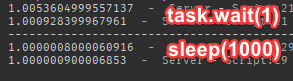# Faster Clock then "while game.RunService.Heaterbeat:Wait()"

I am attempting to create a CPU emulation and for my needs 60 clocks per second would not be fast enough, any ways to create a faster loop?

the only thing I could think of is while wait() do but I’m not sure if its faster. If it isn’t I don’t think there is anything faster.

You can try something like this: (tested in Studio)
It basically works by using `while true do break end`, which should break very quickly and waits around `0.0000001000008`, which is then scaled to milliseconds.

``````local function sleep(t: number)
t = t * .001

local now = os.clock()
local delta

if .02 <= t then
repeat
until os.clock() + delta >= now + t
end

repeat
while true do break end
until now + t <= os.clock()
end
``````

`t` is in milliseconds.

Benchmarks:

Benchmarking code:

``````for _ = 1, 1000, 1 do
local t = os.clock()
sleep(10)
print(os.clock() - t)
end
``````

Another example with `task.wait()` vs `sleep` with waiting one second:Benchmarking code:

``````for _ = 1, 2, 1 do
local t = os.clock()
print(os.clock() - t)
end

print(string.rep("-", 50))

for _ = 1, 2, 1 do
local t = os.clock()
sleep(1000)
print(os.clock() - t)
end
``````
2 Likes

Here is my wait function that is down to 100ns accuracy on my pc.

``````function waitAccurate(ts)
local t = os.clock()
while os.clock()-t < ts do

end
end
``````